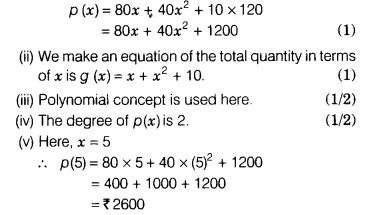# Kishan sells x kg of apple at the rate of ₹ 80 per kg

Kishan sells x kg of apple at the rate of ₹ 80 per kg, some oranges at the rate of ₹ 40 per kg whose quantity is equal to the square of the apple quantity. Along that he sells 10 kg pomegranate at the rate of ₹ 120 per kg.
(i) Write an equation of the total cost of the quantity.
(ii) Write an equation of the total quantity.
(iii) Which mathematical concept is used here?
(iv) Find the degree of an equation of total cost of quantity.
(v) Kishan thinks that he will sell 5 kg apples for ₹ 2600. Is Kishan’s assumption correct? What value is depicted by Kishan?

(i) We make an equation of the total cost of quantity in terms of x isHence, Kishan earns ₹ 2600 by selling 5 kg apples. So, Kishan’s decision is correct.
The value depicted by Kishan’s is smartness.